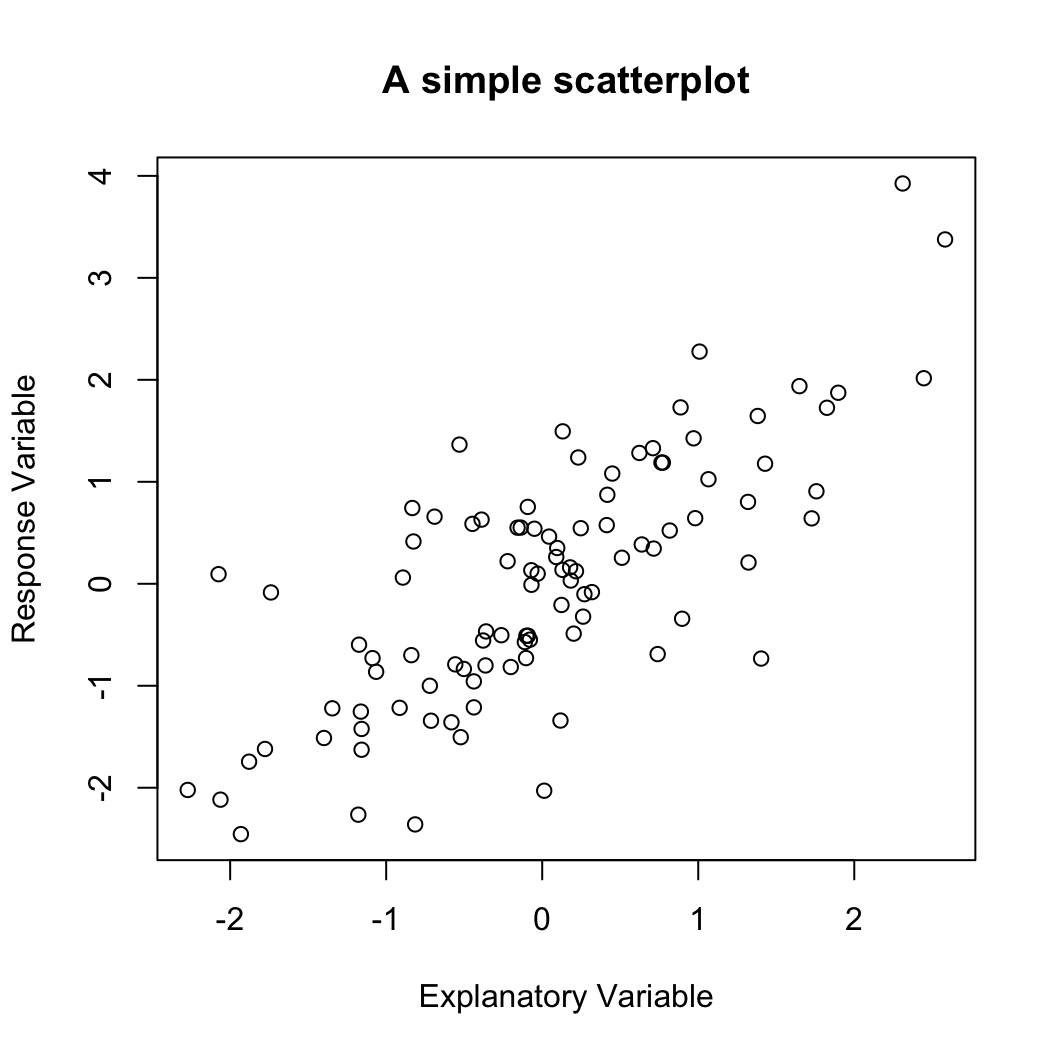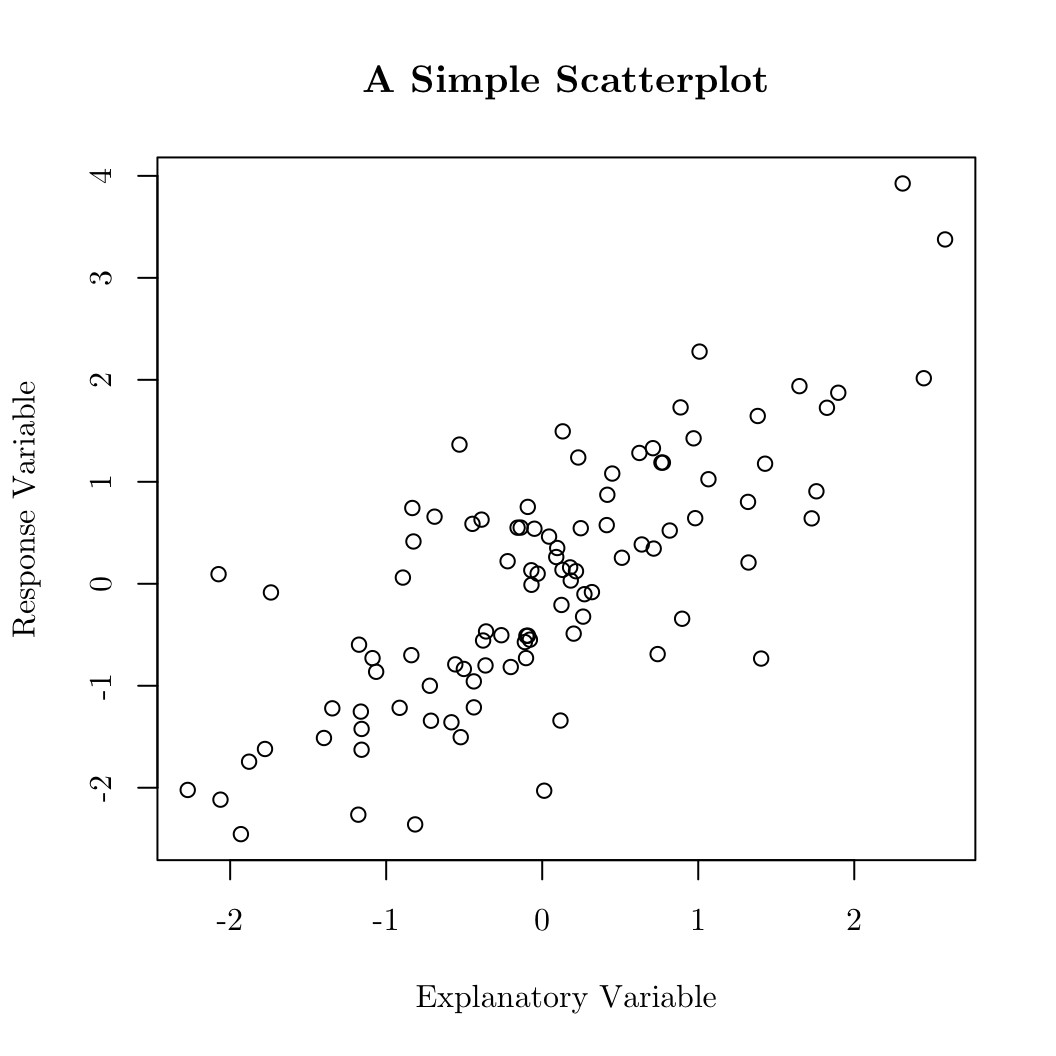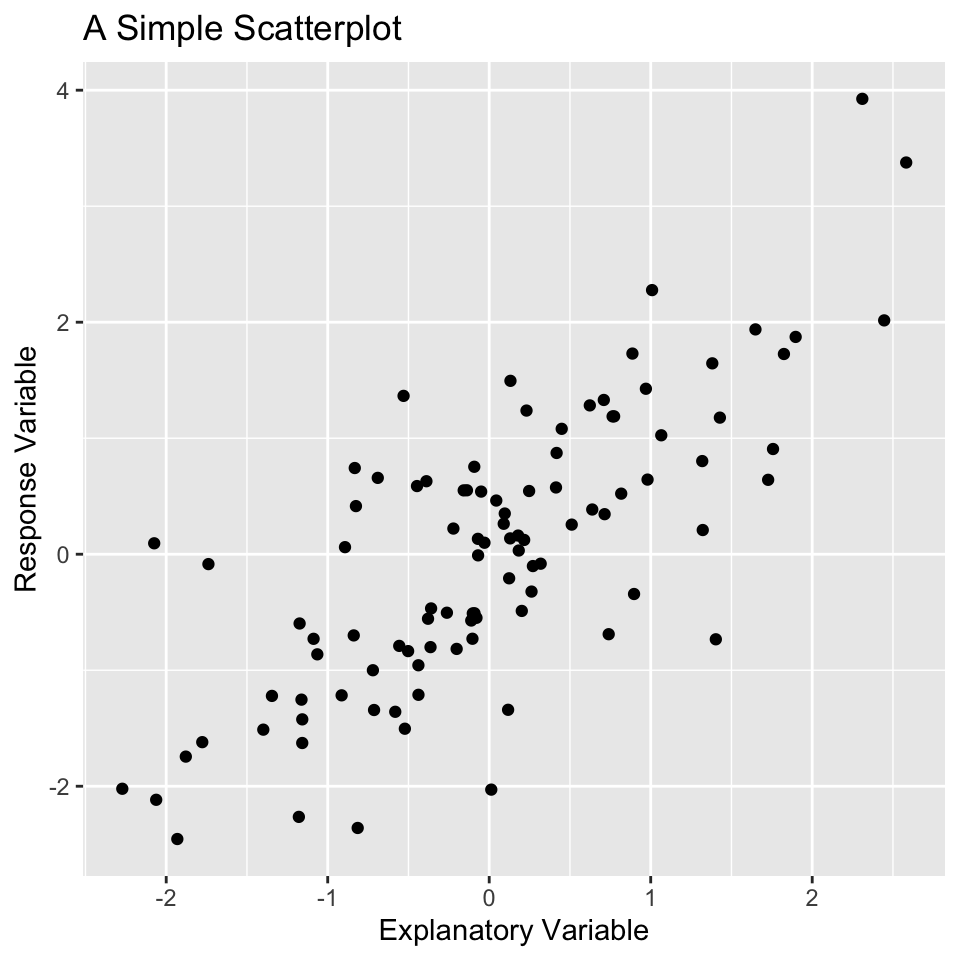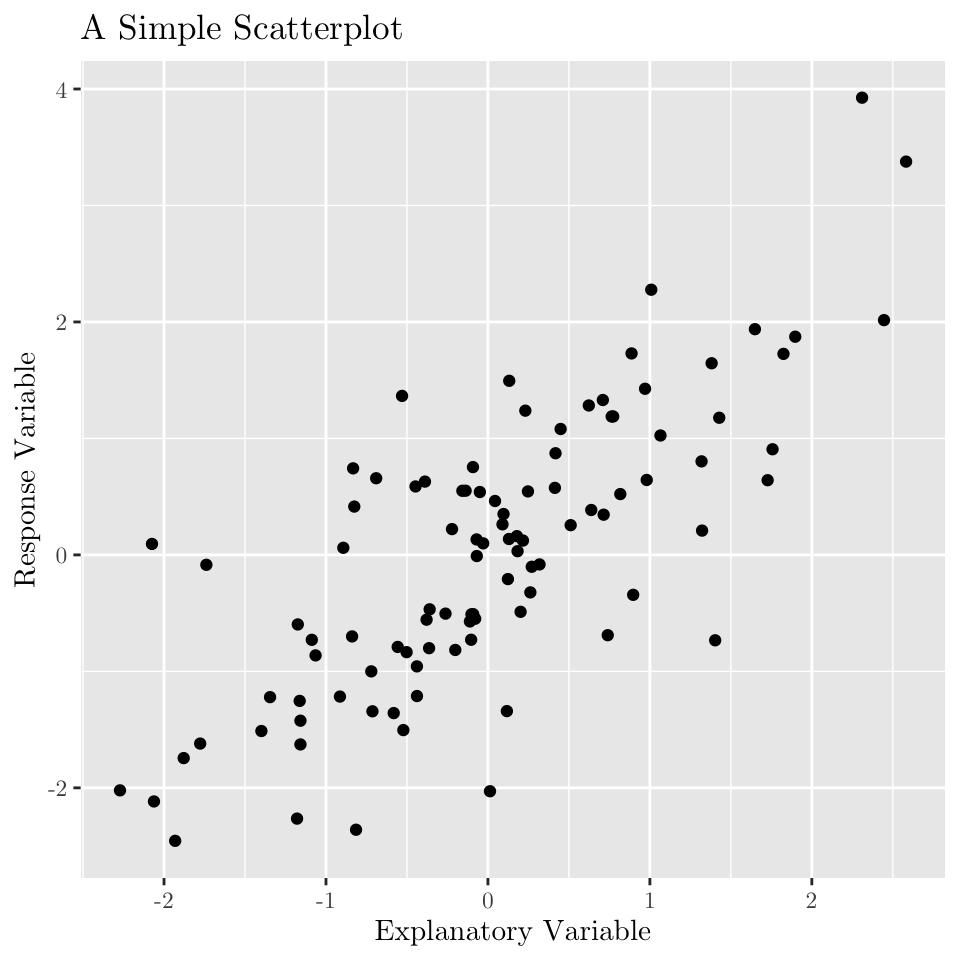# LaTeX Font for Data Viz in R

For future reference

Quang Nguyen https://github.com/qntkhvn
April 21, 2022

So… I had been wondering about how to change the font when making data viz in R to the $$\LaTeX$$ font (Computer Modern). Turns out, it’s not that complicated.

### Set up

(Note: The set up shown below is for a Mac device. I imagine it’d be something similar for Windows.)

• I first downloaded the Computer Modern font (with .ttf file extension) from the internet. It is available on a few different websites, and the one I ended up with was https://www.fontsquirrel.com/fonts/computer-modern.

• I then imported the unzipped folder of the downloaded font file to the Font Book app.

And… the set up is basically it. Now, let’s make some data viz. First, let’s quickly generate some data.

set.seed(100)
x <- rnorm(100)
y <- x + rnorm(100)


I’m going to illustrate this for both base plotting and ggplot2.

### baseR

By default, the font of a base R plot looks like this:

plot(x,
y,
main = "A simple scatterplot",
xlab = "Explanatory Variable",
ylab = "Response Variable")To apply the $$\LaTeX$$ font (or any font you want), use the good ol’ par() function, and specify family = "the font name" inside of par(). Here, the name of the font is “CMU Serif”.

par(family = "CMU Serif")
plot(x,
y,
main = "A Simple Scatterplot",
xlab = "Explanatory Variable",
ylab = "Response Variable")(I’m sure there are other ways of doing this in base R too…)

### ggplot2

For ggplot2, the default theme appears like this:

library(tidyverse)
p <- tibble(x, y) %>%
ggplot(aes(x, y)) +
geom_point() +
labs(x = "Explanatory Variable",
y = "Response Variable",
title = "A Simple Scatterplot")
pWe can then call theme() to customize the text font.

p +
theme(text = element_text(family = "CMU Serif"))Of course, we can also do this separately for the different individual theme components (axis.text, plot.title, etc.) of ggplot2.

Ta-da!

(I haven’t blogged in awhile. This is my first blog since last August, before I moved to Chicago. Feels great to be back blogging again.)

### Citation

Nguyen (2022, April 21). The Q: LaTeX Font for Data Viz in R. Retrieved from https://qntkhvn.netlify.app/posts/2022-04-20-latex-plot/
@misc{nguyen2022latex,
}